## A velocity selector in a mass spectrometer uses a 0.150 T magnetic field. (a) What electric field strength (in volts per meter) is needed to

Question

A velocity selector in a mass spectrometer uses a 0.150 T magnetic field. (a) What electric field strength (in volts per meter) is needed to select a speed of 4.40 ✕ 106 m/s? V/m

in progress 0
2 weeks 2021-09-05T16:26:04+00:00 1 Answers 0 views 0

The electric field strength is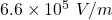Explanation:

Given that,

Magnetic field = 0.150 T

Speed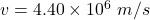We need to calculate the electric field strength

Using formula of velocity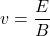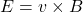Where, v = speed

B = magnetic field

Put the value into the formula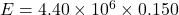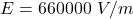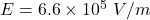Hence, The electric field strength is